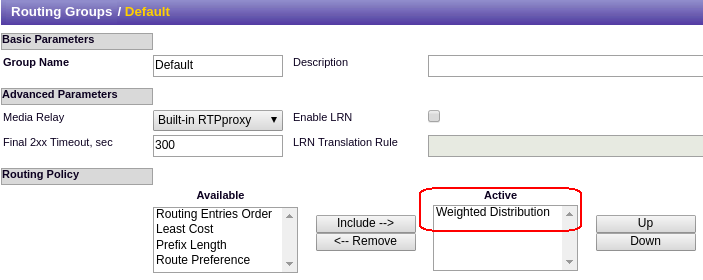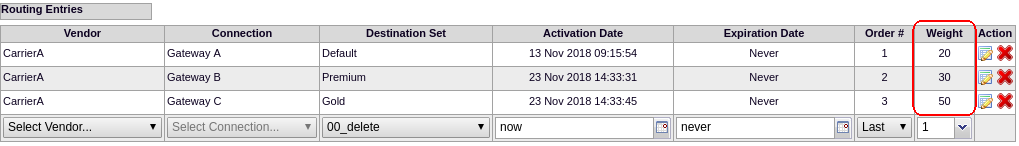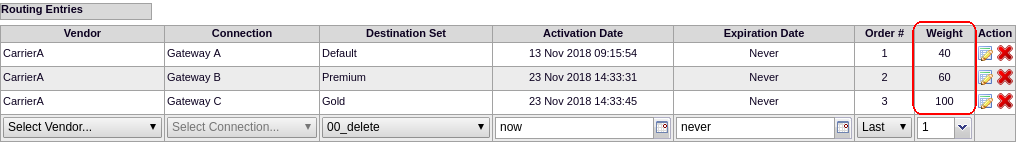## General information

Sometimes you may need to split traffic by a specific rate to different gateways.  For example, you need to route 20% of calls to gateway "X", 30% of calls to gateway "Y" and the remaining 50% of the calls to gateway "Z".  The following example describes how you can configure this:

## Example

• You have 3 gateways and you need to distribute traffic between them
• 20% of traffic should be routed to the 1st gateway
• 30% of traffic should be routed to the 2nd gateway
• 50% of traffic should be routed to the 3rd gateway

In order to achieve this you need to set a Weighted Distribution policy inside a Routing Group:Next, you need to specify the weight for every routing entry according to your requirements:The recommended values for use are the ones which give you 100% in total.  The example above provides the values 20% + 30% + 50% = 100%

## Calculation concepts

The Weight column accepts any value because the probability is calculated by the following formula:This means you can specify even the values 40, 60 and 100 as below:and will result in the same probability:

```100 / 200 *  40 = 20%         (Probability of route #1)
100 / 200 *  60 = 30%         (Probability of route #2)
100 / 200 * 100 = 50%         (Probability of route #3)```

The only difference in the formats is that the values (20, 30, 50) which result in 100% are more easily perceived correctly.  There is no need for the Administrator to do any calculations.

Sometimes, when you use values that don't add up to 100% in total, it might lead you to think that the configuration is incorrect.  For example, you could define the values 35, 45 and 85 expecting they will result in 35%, 45% and 85% probability; but, this would be misleading.  Considering the formula above, the values 35, 45, and 85 will result in 21.2%, 27.2% and 51.5%

## The impact of previous triage results

An important aspect of the Weighted Distribution policy is that in practice, it does not take into account any previous results of the triage of calls routed.

In other words, if you have 100 calls, it is not guaranteed that exactly 20 calls will go to one route, 30 calls to another and the remaining 50 calls to another (as in the main example of this article).  Instead, every new call will participate in the 20/30/50 triage from scratch (without any relation to previous results).